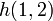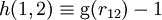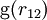# Total correlation function

The total correlation function,$h(1,2)$, is given by$h(1,2) \equiv {\mathrm g}(r_{12}) -1$,
where${\mathrm g}(r_{12})$ is the pair distribution function.